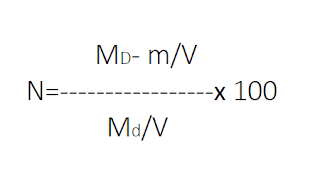Pipette Method - For Sedimentation analysis - Civil Engineering

## Monday, 2 April 2018

### Pipette Method - For Sedimentation analysis

Pipette is a simple apparatus made of glass. It is used for drawing samples of soil suspension.
A suitable amount of oven-dried sample is mixed with distilled water. A suitable quantity of deflocculating agent is added to it. The mixture is then thoroughly shaken and placed in a jar. The suspension is allowed to set down for settlement.

As soon as the particles start settling, a stopwatch is started. By immersing the pipette at a known depth (He), soil water suspension samples are taken out at a regular interval.
The exact volume of sample collected, corresponding time interval and He are recorded. The sample thus collected is then put in an oven for removing water.

### Pipette Method - Equation Part

Let,
M= Total dry mass of the soil used in the suspension.
m = Mass of dispersing agent present in the total suspension of volume
V =Volume of total suspension.
M= Dry mass per ml of all the soil particles in suspension collected by pipette.
Then the percentage finer (N) than the diameter size D is given by the expression:Pipette Method
The diameter (D) of the largest particles present in the sample is calculated from the following equation.

D = 18η Hew(G-1)t

Where D=Diameter of the spherical particle in m.
η = Viscosity of the liquid in kN-s / m2 .
G = Specific gravity of the solid soil.
He = Effective depth in m.
t = Time of sampling after the start of settlement in sec.
γw = Unit weight of the liquid in kN / m3

Thus, with the help of equation (1) and (2) we can get various diameter D and the percentage of particles finer(N%) than this diameter.

The pipette method can be used only in the laboratory and the apparatus used is costly and sensitive. It also requires more time and is not suitable for routine control tests.